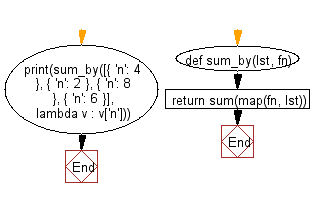﻿ Python: Calculate the sum of a list, after mapping each element to a value using a given function - w3resource# Python: Calculate the sum of a list, after mapping each element to a value using a given function

## Python List: Exercise - 250 with Solution

Write a Python program to calculate the sum of a list, after mapping each element to a value using the provided function.

• Use map() with fn to map each element to a value using the provided function.
• Use sum() to return the sum of the values.

Sample Solution:

Python Code:

``````def sum_by(lst, fn):
return sum(map(fn, lst))
print(sum_by([{ 'n': 4 }, { 'n': 2 }, { 'n': 8 }, { 'n': 6 }], lambda v : v['n']))
```
```

Sample Output:

```20
```

Flowchart:## Visualize Python code execution:

The following tool visualize what the computer is doing step-by-step as it executes the said program:

Python Code Editor:

Have another way to solve this solution? Contribute your code (and comments) through Disqus.

What is the difficulty level of this exercise?

Test your Python skills with w3resource's quiz

﻿

## Python: Tips of the Day

Floor Division:

When we speak of division we normally mean (/) float division operator, this will give a precise result in float format with decimals.

For a rounded integer result there is (//) floor division operator in Python. Floor division will only give integer results that are round numbers.

```print(1000 // 300)
print(1000 / 300)```

Output:

```3
3.3333333333333335```